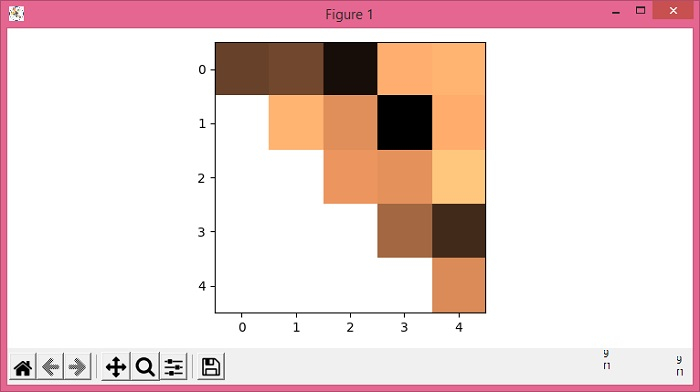# Plotting only the upper/lower triangle of a heatmap in Matplotlib

MatplotlibPythonData Visualization

To plot only the upper/lower triangle of a heatmap in matplotlib, we can use numpy to get the masked 2D array and convert them into an image to produce a heatmap.

## Steps

• Set the figure size and adjust the padding between and around the subplots.

• Create a random data of 5×5 dimension.

• Use numpy.tri() method to create an array with 1's at and below the given diagonal and 0's elsewhere.

• Get the masked 2D array data with masked array (Using step 3).

• Use imshow() method to display the data as an image, i.e., on a 2D regular raster.

• To display the figure, use show() method.

## Example

import numpy as np
from matplotlib import pyplot as plt
plt.rcParams["figure.figsize"] = [7.50, 3.50]
plt.rcParams["figure.autolayout"] = True
data = np.random.rand(5, 5)
plt.show()